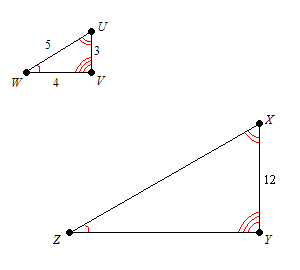# Similar Triangles

Two triangles are said to be similar if their corresponding angles are congruent and the corresponding sides are in proportion . In other words, similar triangles are the same shape, but not necessarily the same size.

The triangles are congruent if, in addition to this, their corresponding sides are of equal length.

The side lengths of two similar triangles are proportional. That is, if $\Delta UVW$ is similar to $\Delta XYZ$ , then the following equation holds:

$\frac{UV}{XY}=\frac{UW}{XZ}=\frac{VW}{YZ}$

This common ratio is called the scale factor .

The symbol $\sim$ is used to indicate similarity.

Example:

$\Delta UVW\sim \Delta XYZ$ . If $UV=3,VW=4,UW=5\text{\hspace{0.17em}}\text{\hspace{0.17em}}\text{and}\text{\hspace{0.17em}}\text{\hspace{0.17em}}XY=12$ , find $XZ$ and $YZ$ .

Draw a figure to help yourself visualize.Write out the proportion. Make sure you have the corresponding sides right.

$\frac{3}{12}=\frac{5}{XZ}=\frac{4}{YZ}$

The scale factor here is $\frac{3}{12}=\frac{1}{4}$ .

Solving these equations gives $XZ=20$ and $YZ=16$ .

The concepts of similarity and scale factor can be extended to other figures besides triangles.